# Slope Geometry

The Slope Geometry parameters for your RocTopple model are entered in the Geometry tab of the Input Data dialog. To open the tab:

• Select Input Dataon the toolbar or the Analysis menu or press F3.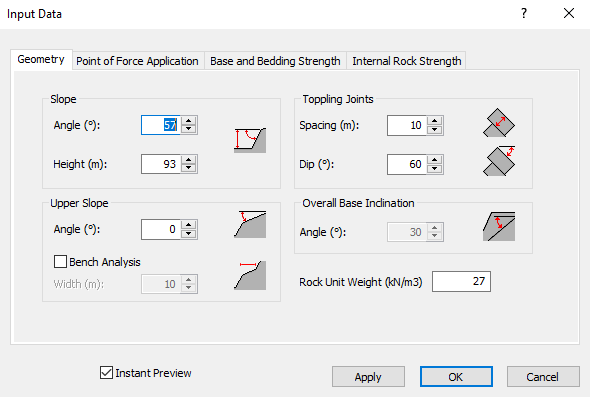You can enter the following data in the tab:

• Slope Angle
• Slope Height
• Upper Slope Angle
• Bench Analysis Width
• Toppling Joints Spacing
• Toppling Joints Dip
• Overall Base Inclination Angle
• Rock Unit Weight

## Slope Angle and Height

The Slope Angle is the dip angle of the front face of the slope. The Slope Height is the vertical height of the slope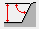measured from the toe to the crest.

## Upper Slope Angle

The Upper Slope Angle is the dip angle of the upper face of the slope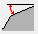. If horizontal, enter zero. A negative value can also be entered if the upper slope dips backwards in the opposite direction from the front slope face.

## Bench Analysis Width

By default RocTopple, automatically computes a maximum Bench Width based on the slope geometry (Slope Height, Slope Angle, Upper Slope Angle, Overall Base Inclination Angle). This determines the maximum possible bench width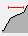(i.e., horizontal extent of the upper slope).

If you want to define a Bench Width that is smaller than the default, select the Bench Analysis check box and enter a value of Bench Width. In this case, the number of toppling blocks is automatically truncated to approximate the required Bench Width. The Bench Width number is not exact, it is simply used to reduce the number of toppling blocks on the upper slope. The actual Bench Width greater than or equal to (not less than) the number you enter due to the discrete number of blocks. The figure below shows a model with a user-defined Bench Width.NOTE: The Bench Width is defined as the horizontal distance from the crest of the slope, even if the Upper Slope Angle is not horizontal.

## Toppling Joint Spacing and Dip

The Toppling Joint Dip is the dip angle into the slope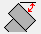of the primary toppling discontinuities. The Toppling Joint Spacing is the perpendicular spacing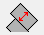between toppling discontinuities. In RocTopple version 1.0, it is assumed that the spacing is equal for all toppling blocks.

## Overall Base Inclination

The Overall Base Inclinationis the angle of a line from the toe of the slope to the exit point on the upper slope, which defines the overall failure direction or base line of the toppling mass.

In general, the Overall Base Inclination is NOT equal to the inclination of the base of the toppling blocks. Because it is assumed that the base of each toppling block is perpendicular to the toppling joint angle, the Overall Base Inclination is always greater than (or equal to) the block base angle.

The Overall Base Inclination is equal to the block base angle only when the toppling joint dip is perpendicular to the overall base inclination (i.e. if toppling joint dip + overall base inclination = 90 degrees).

## Rock Unit Weight

The Rock Unit Weight is entered according to the unit system specified in the Project Settings dialog.